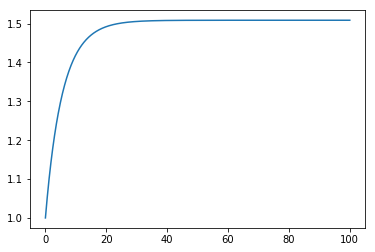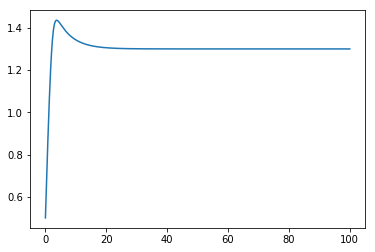We covered the idea of simulating an arbitrary transfer function system in a previous notebook. What happens if we have to simulate a controller (specified as a transfer function) and a system specified by differential equations together?

# 91. Nonlinear tank system¶

Let’s take the classic tank system, with a square root flow relationship on the outflow and a nonlinear valve relationship.\begin{align} \frac{dV}{dt} &= (F_{in} - F_{out}) \\ h &= \frac{V}{A} \\ f(x) &= \alpha^{x - 1} \\ F_{out} &= K f(x) \sqrt{h} \\ \end{align}
:

import numpy
import matplotlib.pyplot as plt
%matplotlib inline


Parameters

:

A = 2
alpha = 20
K = 2


Initial conditions (notice I’m not starting at steady state)

:

Fin = 1
h = 1
V = A*h
x0 = x = 0.7


Valve characterisitic

:

def f(x):
return alpha**(x - 1)


Integration time

:

ts = numpy.linspace(0, 100, 1000)
dt = ts


Notice that I have reordered the equations here so that they can be evaluated in order to find the volume derivative.

:

hs = []
for t in ts:
h = V/A
Fout = K*f(x)*numpy.sqrt(h)
dVdt = Fin - Fout
V += dVdt*dt

hs.append(h)

:

plt.plot(ts, hs)

:

[<matplotlib.lines.Line2D at 0x10e93ca20>]# 92. PI Control¶

Now we can include a controller controlling the level by manipulating the valve fraction

:

import scipy.signal


Let’s do a PI controller:

$G_c = K_c(1 + \frac{1}{\tau_I s}) = \frac{K_C \tau_I s + K_Cs^0}{\tau_I s + 0s^0}$
:

Kc = -1
tau_i = 5


In versions of scipy < 1.0, Gc would automatically have a .A attribute. After 1.0, we need to convert to state space explicitly with .to_ss().

:

Gc = scipy.signal.lti([Kc*tau_i, Kc], [tau_i, 0]).to_ss()

:

hsp = 1.3

:

V = 1
hs = []
xc = numpy.zeros([Gc.A.shape, 1])
for t in ts:
h = V/A
e = hsp - h  # we cheat a little here - the level we use to calculate the error is from the previous time step

# e is in the input to the controller, yc is the output
dxcdt = Gc.A.dot(xc) + Gc.B.dot(e)
yc = Gc.C.dot(xc) + Gc.D.dot(e)

x = x0 + yc[0,0]  # x0 is the controller bias

Fout = K*f(x)*numpy.sqrt(h)
dVdt = Fin - Fout

V += dVdt*dt
xc += dxcdt*dt

hs.append(h)

:

plt.plot(ts, hs)

:

[<matplotlib.lines.Line2D at 0x1c1a0f31d0>]